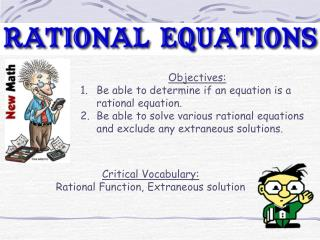DownloadDownload PresentationObjectives: Be able to determine if an equation is a rational equation.

# Objectives: Be able to determine if an equation is a rational equation.

Télécharger la présentation## Objectives: Be able to determine if an equation is a rational equation.

- - - - - - - - - - - - - - - - - - - - - - - - - - - E N D - - - - - - - - - - - - - - - - - - - - - - - - - - -
##### Presentation Transcript

1. Objectives: • Be able to determine if an equation is a rational equation. • Be able to solve various rational equations and exclude any extraneous solutions. Critical Vocabulary: Rational Function, Extraneous solution

2. I. Rational Functions A rational equation is an equation that contains rational expressions (x in the denominator) Formal Definition: A rational Function is a ratio of two polynomials written in the form No, that would be a linear equation. Looks like you got it. I think I get it. I bet this is a rational equation. What is a Rational Equation? In Simple terms, it’s a fraction. So, would this equation be rational? So, what is a rational then?

3. II. Solving Rational Equations a. Solving a Rational: Cross Multiplication (Proportion) 1. First determine what “x” can’t be (6t + 7)(2t - 4) = (4t - 1)(3t + 8) Cross Multiply 12t2 - 10t - 28 = 12t2 + 29t - 8 FOIL -10t - 28 = 29t - 8 What are talking about? What is an extraneous solution? -39t - 28 = -8 -39t = 20 Solution: x = -20/39 That’s where your solution is one of the values that “x” can’t be. Look like you didn’t get any extraneous solutions.

4. II. Solving Rational Equations b. Solving a Rational: By Finding LCM (Denominator) 2. What can x not be? Multiply by LCD Distribute 6 + 8x = 7 8x = 1 This is not an extraneous solution either. Solution: x = 1/8 No….really?

5. II. Solving Rational Equations 3. What can x not be? Multiply by LCD Distribute 3x + 2(x - 1) = 3 3x + 2x - 2 = 3 This means there is no solution 5x - 2 = 3 5x = 5 x = 1 This is extraneous. What does that mean? Solution: None

6. II. Solving Rational Equations 4. Factor Denominators 2x = 4 - (x - 2) 2x = 4 - x + 2 3x = 6 x = 2 Solution: None

7. Page 593 #5 - 27 odd